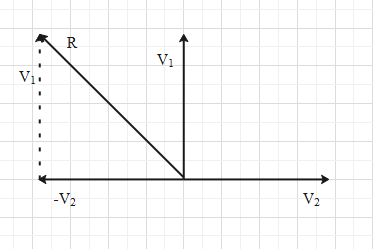QuestionAnswers

# The second’s hand of a watch has a length 6cm. Speed of end point and magnitude of difference of velocities at two perpendicular positions will be$\text{A}\text{. }2\pi mm/s,0mm/s$$\text{B}\text{. }2\sqrt{2}\pi mm/s,4.44mm/s$$\text{C}\text{. }2\sqrt{2}\pi mm/s,2\pi mm/s$$\text{A}\text{. }2\pi mm/s,2\sqrt{2}\pi mm/s$Verified
131.4k+ views
Hint: First find the angular velocity of the second’s hand knowing that it completes one full rotation in 60 seconds. Then use the formula $v=r\omega$ and find the velocity of the end point. Using the same formula, find the velocities of the end points at two perpendicular positions. Then form vectors analysis, find the magnitude of the difference.

Formula used:
$\omega =\dfrac{\theta }{t}$
$v=r\omega$

Since the second’s hand of a watch is in rotational motion, we have to use rotational motion to solve the question.
As we said that the second’s is under rotational motion, let us calculate first its angular velocity. Angular velocity is the angle by which the second’s hand rotates in one unit of time. Let the angular velocity of the second’s hand be $\omega$.
We know that the second’s hand of a watch rotates for an angle of 2$\pi$ radians in one minute. One minute is equal to 60 seconds.
Therefore, the angular velocity of the hand is $\omega =\dfrac{2\pi }{60}=\dfrac{\pi }{30}{{s}^{-1}}$.
When the second’s hand is rotating with this angular velocity, any point on the hand has a velocity perpendicular to the hand. The velocity of a point on the hand at a distance r from the point of rotation will be $v=r\omega$ … (i).
We have to find the speed of the end point. Therefore, r will be equal to the length of the hand. i.e. r=6cm.
Substitute the values of r and $\omega$ in equation (i).
$\Rightarrow v=6\left( \dfrac{\pi }{30} \right)=\dfrac{\pi }{5}cm/s=\dfrac{\pi }{5}\left( 10mm/s \right)=2\pi mm/s$
Therefore, the speed of the end point is $2\pi mm/s$.
Now, let consider any two perpendicular positions of the second’s hand. Say one is pointing 12 and the other is pointing 3 of the watch.
The speed of the end point at these positions will be equal to $2\pi mm/s$.
But the directions of the velocities will be different. Let velocity when the hand is pointing 12 be $\overrightarrow{{{V}_{1}}}$ and the velocity at the other position be $\overrightarrow{{{V}_{2}}}$. The directions of these velocities are as shown below.Now we have to find the magnitude of the resultant of $\overrightarrow{{{V}_{1}}}-\overrightarrow{{{V}_{2}}}$ .
The resultant of $\overrightarrow{{{V}_{1}}}-\overrightarrow{{{V}_{2}}}$ i.e. $\overrightarrow{R}$ can be understood from the figure below.
From the figure we get that the magnitude of $\overrightarrow{R}$ is $R=\sqrt{V_{1}^{2}+V_{2}^{2}}$ ….. (ii)
And we know that ${{V}_{1}}={{V}_{2}}=2\pi mm/s$.
Substitute the values of ${{V}_{1}}$ and ${{V}_{2}}$ in equation (ii).
$\Rightarrow R=\sqrt{{{\left( 2\pi \right)}^{2}}+{{\left( 2\pi \right)}^{2}}}=\sqrt{2{{\left( 2\pi \right)}^{2}}}=2\pi \sqrt{2}mm/s$
This means that the magnitude of difference between velocities at any two perpendicular position is $2\pi \sqrt{2}mm/s$.

Hence, the correct option is D.